×#### Thank you for registering.

One of our academic counsellors will contact you within 1 working day.

Click to Chat

1800-1023-196

+91-120-4616500

CART 0

• 0

MY CART (5)

Use Coupon: CART20 and get 20% off on all online Study Material

ITEM
DETAILS
MRP
DISCOUNT
FINAL PRICE
Total Price: Rs.

There are no items in this cart.
Continue Shopping• Complete JEE Main/Advanced Course and Test Series
• OFFERED PRICE: Rs. 15,900
• View Details

```Chapter 15: Properties of Triangles Exercise – 15.1

Question: 1Take three non-collinear points A. B and C on a page of your notebook. Join AB, BC and CA. What figure do you get? Name the triangle. Also, name

(i) The side opposite to ∠B

(ii) The angle opposite to side AB

(iii) The vertex opposite to side BC

(iv) The side opposite to vertex B.

Solution:

(i) AC

(ii) ∠B

(iii) A

(iv) AC

Question: 2

Take three collinear points A, B and C on a page of your note book. Join AB. BC and CA. Is the figure a triangle? If not, why?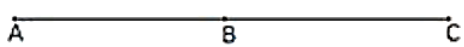Solution:

No, the figure is not a triangle. By definition a triangle is a plane figure formed by three non-parallel line segments

Question: 3

Distinguish between a triangle and its triangular region.

Solution:

A triangle is a plane figure formed by three non-parallel line segments, whereas, its triangular region includes the interior of the triangle along with the triangle itself.

Question: 4

D is a point on side BC of a ∆CAD is joined. Name all the triangles that you can observe in the figure. How many are they?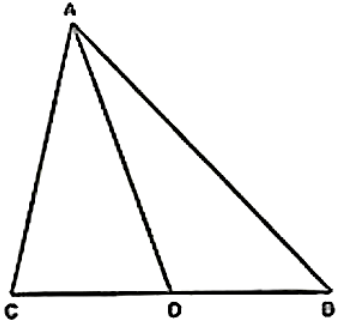Solution:

We can observe the following three triangles in the given figure

(i) ∆ABC

(ii) ∆ACD

Question: 5

A, B. C and D are four points, and no three points are collinear. AC and BD intersed at O. There are eight triangles that you can observe. Name all the trianglesSolution:

(i) ∆ABC

(ii) ∆ABD

(iii) ∆ABO

(iv) ∆BCD

(v) ∆DCO

(vi) ∆AOD

(vii) ∆ACD

(viii) ∆BCD

Question: 6

What is the difference between a triangle and triangular region?

Solution:

Plane of figure formed by three non-parallel line segments is called a triangle where as triangular region is the interior of triangle ABC together with the triangle ABC itself is called the triangular region ABC

Question: 7

Explain the following terms:

(i) Triangle

(a) Parts or elements of a triangle

(iii) Scalene triangle

(iv) Isosceles triangle

(v) Equilateral triangle

(vi) Acute triangle

(vii) Right triangle

(viii) Obtuse triangle

(ix) Interior of a triangle

(x) Exterior of a triangle

Solution:

(i) A triangle is a plane figure formed by three non-parallel line segments.

(ii) The three sides and the three angles of a triangle are together known as the parts or elements of that triangle.

(iii) A scalene triangle is a triangle in which no two sides are equal.(iv) An isosceles triangle is a triangle in which two sides are equal. Isosceles triangle(v) An equilateral triangle is a triangle in which all three sides are equal. Equilateral triangle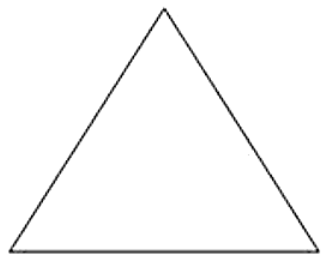(vi) An acute triangle is a triangle in which all the angles are acute (less than 90°).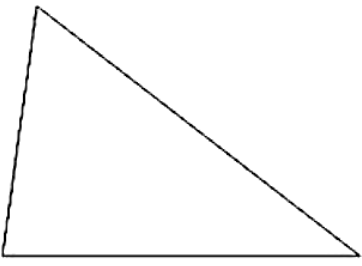(vii) A right angled triangle is a triangle in which one angle is right angled, i.e. 90°.(viii) An obtuse triangle is a triangle in which one angle is obtuse (more than 90°).(ix) The interior of a triangle is made up of all such points that are enclosed within the triangle.

(x) The exterior of a triangle is made up of all such points that are not enclosed within the triangle.

Question: 8

In Figure, the length (in cm) of each side has been indicated along the side. State for each triangle angle whether it is scalene, isosceles or equilateral: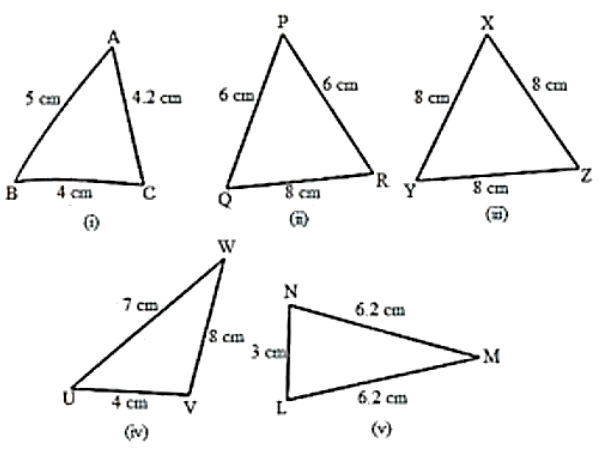Solution:

(i) This triangle is a scalene triangle because no two sides are equal.

(ii) This triangle is an isosceles triangle because two of its sides, viz. PQ and PR, are equal.

(iii) This triangle is an equilateral triangle because all its three sides are equal.

(iv) This triangle is a scalene triangle because no two sides are equal.

(v) This triangle is an isosceles triangle because two of its sides are equal.

Question: 9

There are five triangles. The measures of some of their angles have been indicated. State for each triangle whether it is acute, right or obtuse.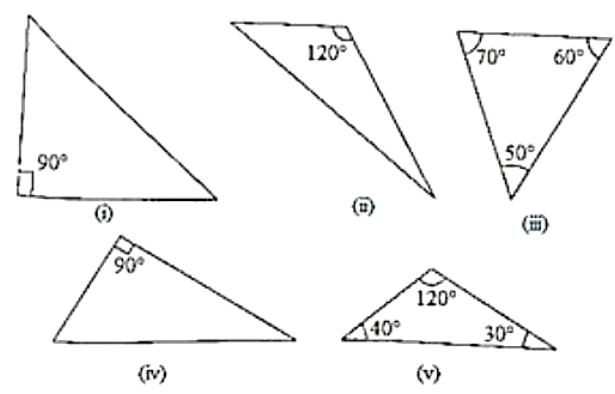Solution:

(i) This is a right triangle because one of its angles is 90°.

(ii) This is an obtuse triangle because one of its angles is 120°, which is greater than 90°.

(iii) This is an acute triangle because all its angles are acute angles (less than 90°).

(iv) This is a right triangle because one of its angles is 90°.

(v) This is an obtuse triangle because one of its angles is 110°, which is greater than 90°.

Question: 10

Fill in the blanks with the correct word/symbol to make it a true statement:

(i) A triangle has _____ sides.

(ii) A triangle has ______ vertices.

(iii) A triangle has _____ angles.

(iv) A triangle has ________ parts.

(v) A triangle whose no two sides are equal is known as ________

(v0 A triangle whose two sides are equal is known as ________

(vii) A triangle whose all the sides are equal is known as ________

(viii) A triangle whose one angle is a right angle is known as _________

(ix) A triangle whose all the angles are of measure less than 90' is known as _________

(x) A triangle whose one angle is more than 90' is known as _________

Solution:

(i) three

(ii) three

(iii) three

(iv) six (three sides + three angles)

(v) a scalene triangle

(vi) an isosceles triangle

(vii) an equilateral triangle

(viii) a right triangle

(ix) an acute triangle

(x) an obtuse triangle

Question: 11

In each of the following, state if the statement is true (T) or false (F):

(i) A triangle has three sides.

(ii) A triangle may have four vertices.

(iii) Any three line-segments make up a triangle.

(iv) The interior of a triangle includes its vertices.

(v) The triangular region includes the vertices of the corresponding triangle.

(vi) The vertices of a triangle are three collinear points.

(vii) An equilateral triangle is isosceles also.

(viii) Every right triangle is scalene.

(ix) Each acute triangle is equilateral.

(x) No isosceles triangle is obtuse.

Solution:

(i) True.

(ii) False. A triangle has three vertices.

(iii) False. Any three non-parallel line segments can make up a triangle.

(iv) False. The interior of a triangle is the region enclosed by the triangle and the vertices are not enclosed by the triangle.

(v) True. The triangular region includes the interior region and the triangle itself.

(vi) False. The vertices of a triangle are three non-collinear points.

(vii) True. In an equilateral triangle, any two sides are equal.

(viii) False. A right triangle can also be an isosceles triangle.

(ix) False. Each acute triangle is not an equilateral triangle, but each equilateral triangle is an acute triangle.

(x) False. An isosceles triangle can be an obtuse triangle, a right triangle or an acute triangle
```### Course Features

• 728 Video Lectures
• Revision Notes
• Previous Year Papers
• Mind Map
• Study Planner
• NCERT Solutions
• Discussion Forum
• Test paper with Video Solution